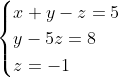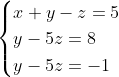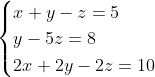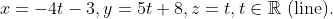## System of Linear Equations – Geometrical Meaning

### Review Terms

linear equation is an equation where the unknowns or variables are powers with exponent one.

For example, 3x – 4y + 5z = 3 is a linear equation because the variables xyz are linear, but xy + 3z = 7 is not linear because of the term xy, which is a product of two variables.

### Geometric Meaning of a Linear Equation

A linear equation ax + by = c, represented in the rectangular coordinates is a line with slope -a/c, while a linear equation of three variables, ax + by + cz = d, is a plane in space, whose normal vector is <a, b, c>.

When we solve a system of linear equations, we look for a solution that verifies all equations in the system, or the solution is at the intersection of all equations.

### Geometric Meaning of a System of Linear Equations

Therefore, a system of two linear equations with two unknowns represents the intersection of the two lines in a plane, and a system of three linear equations with three unknowns represents the intersection of three planes in space.

Because the intersection of three planes in space could be a point (when the three planes intersect in a single point), one line (when all three planes contain the line), one plane (when all three planes are identical), or empty set (when there is no common intersection to all three planes).

Similarly, we have three types of solutions to a system of three equations: unique, infinitely many, or no solution.

### Application

For each of the systems of equations, determine and give the geometric meaning (if any) of the solution.

1.2.3.### Solutions

1. Using back substitution, we obtain the unique solution x = 1y = 3z = -1, which is a point.

2. No solution. The last two equations are inconsistent.

3. Infinitely many solutions, the first and third equations are equivalent.